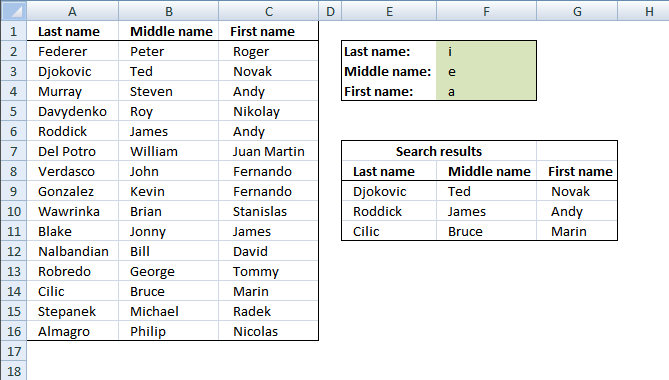Author: Oscar Cronquist Article last updated on November 23, 2021

###Question:
Can expand this equation set into more than two colums of data, say if I had a first, middle and last name column could I only display the values in which all three cases are true?This blog article answers a question in this article: Lookup with multiple criteria and display multiple search results using excel formula

Array formula in F8:

=INDEX(\$B\$3:\$D\$17, SMALL(IF(MMULT(--(ISNUMBER(SEARCH(TRANSPOSE(\$G\$2:\$G\$4), \$B\$3:\$D\$17))), {1; 1; 1})=3, MATCH(ROW(\$B\$3:\$D\$17), ROW(\$B\$3:\$D\$17)), ""), ROWS(\$A\$1:A1)), COLUMNS(\$A\$1:A1))

To enter an array formula, type the formula in a cell then press and hold CTRL + SHIFT simultaneously, now press Enter once. Release all keys.

The formula bar now shows the formula with a beginning and ending curly bracket telling you that you entered the formula successfully. Don't enter the curly brackets yourself.

### Explaining formula in cell F8

#### Step 1 - Search for criteria

The SEARCH function allows you to find a string in a cell and it's position. It also allows you to search for multiple strings in multiple cells if you arrange values in a way that works. That is why I use the TRANSPOSE function to transpose the values.

SEARCH(TRANSPOSE(\$G\$2:\$G\$4), \$B\$3:\$D\$17)

becomes

SEARCH(TRANSPOSE({"a"; "o"; "o"}), \$B\$3:\$D\$17)

becomes

SEARCH(TRANSPOSE({"a"; "o"; "o"}), {"Federer", "Peter", " Roger ";"Djokovic", "Ted", " Novak ";"Murray", "Steven", " Andy ";"Davydenko", "Roy", " Nikolay ";"Roddick", "James", " Andy ";"Del Potro", "William", " Juan Martin ";"Verdasco", "John", " Fernando ";"Gonzalez", "Kevin", " Fernando ";"Wawrinka", "Brian", " Stanislas ";"Blake", "Ted", " James ";"Nalbandian", "Bill", " David ";"Robredo", "George", " Tommy ";"Cilic", "Bruce", " Marin ";"Stepanek", "Michael", " Radek ";"Almagro", "Pihilip", " Nicolas "})

and returns

{#VALUE!, #VALUE!, 3;#VALUE!, #VALUE!, 3;5, #VALUE!, #VALUE!;2, 2, 5;#VALUE!, #VALUE!, #VALUE!;#VALUE!, #VALUE!, #VALUE!;5, 2, 9;5, #VALUE!, 9;2, #VALUE!, #VALUE!;3, #VALUE!, #VALUE!;2, #VALUE!, #VALUE!;#VALUE!, 3, 3;#VALUE!, #VALUE!, #VALUE!;5, #VALUE!, #VALUE!;1, #VALUE!, 5}.

#### Step 2 - Convert numbers to true

The ISNUMBER function returns TRUE if value is a number and FALSE for everything else even errors which is very handy in this case, the search function returns #VALUE! error if a string is not found in a particular cell.

--(ISNUMBER(SEARCH(TRANSPOSE(\$G\$2:\$G\$4), \$B\$3:\$D\$17)))

becomes

--(ISNUMBER({#VALUE!, #VALUE!, 3;#VALUE!, #VALUE!, 3;5, #VALUE!, #VALUE!;2, 2, 5;#VALUE!, #VALUE!, #VALUE!;#VALUE!, #VALUE!, #VALUE!;5, 2, 9;5, #VALUE!, 9;2, #VALUE!, #VALUE!;3, #VALUE!, #VALUE!;2, #VALUE!, #VALUE!;#VALUE!, 3, 3;#VALUE!, #VALUE!, #VALUE!;5, #VALUE!, #VALUE!;1, #VALUE!, 5}))

becomes

--({FALSE, FALSE, TRUE;FALSE, FALSE, TRUE;TRUE, FALSE, FALSE;TRUE, TRUE, TRUE;FALSE, FALSE, FALSE;FALSE, FALSE, FALSE;TRUE, TRUE, TRUE;TRUE, FALSE, TRUE;TRUE, FALSE, FALSE;TRUE, FALSE, FALSE;TRUE, FALSE, FALSE;FALSE, TRUE, TRUE;FALSE, FALSE, FALSE;TRUE, FALSE, FALSE;TRUE, FALSE, TRUE})

The MMULT function can't work with boolean values so we need to convert them into their numerical equivalents. TRUE - 1 annd FALSE - 0 (zero).

--({FALSE, FALSE, TRUE;FALSE, FALSE, TRUE;TRUE, FALSE, FALSE;TRUE, TRUE, TRUE;FALSE, FALSE, FALSE;FALSE, FALSE, FALSE;TRUE, TRUE, TRUE;TRUE, FALSE, TRUE;TRUE, FALSE, FALSE;TRUE, FALSE, FALSE;TRUE, FALSE, FALSE;FALSE, TRUE, TRUE;FALSE, FALSE, FALSE;TRUE, FALSE, FALSE;TRUE, FALSE, TRUE})

and returns

{0, 0, 1;0, 0, 1;1, 0, 0;1, 1, 1;0, 0, 0;0, 0, 0;1, 1, 1;1, 0, 1;1, 0, 0;1, 0, 0;1, 0, 0;0, 1, 1;0, 0, 0;1, 0, 0;1, 0, 1}

#### Step 3 - Sum values row-wise

MMULT(--(ISNUMBER(SEARCH(TRANSPOSE(\$G\$2:\$G\$4), \$B\$3:\$D\$17))), {1;1;1})

becomes

MMULT({0, 0, 1;0, 0, 1;1, 0, 0;1, 1, 1;0, 0, 0;0, 0, 0;1, 1, 1;1, 0, 1;1, 0, 0;1, 0, 0;1, 0, 0;0, 1, 1;0, 0, 0;1, 0, 0;1, 0, 1}, {1;1;1})

and returns

{1; 1; 1; 3; 0; 0; 3; 2; 1; 1; 1; 2; 0; 1; 2}

#### Step 4 - Convert non-numerical values to corresponding row numbers

The following IF function returns the row number if number is 3, there are three strings that must match. FALSE returns "" (nothing).

IF(MMULT(--(ISNUMBER(SEARCH(TRANSPOSE(\$G\$2:\$G\$4), \$B\$3:\$D\$17))), {1; 1; 1})=3, MATCH(ROW(\$B\$3:\$D\$17), ROW(\$B\$3:\$D\$17)), "")

becomes

IF({1; 1; 1; 3; 0; 0; 3; 2; 1; 1; 1; 2; 0; 1; 2}=3, MATCH(ROW(\$B\$3:\$D\$17), ROW(\$B\$3:\$D\$17)), "")

becomes

IF({1; 1; 1; 3; 0; 0; 3; 2; 1; 1; 1; 2; 0; 1; 2}=3, {1; 2; 3; 4; 5; 6; 7; 8; 9; 10; 11; 12; 13; 14; 15}, "")

and returns

{"";"";"";4;"";"";7;"";"";"";"";"";"";"";""}.

#### Step 5 - Extract k-th smallest value in array

The SMALL function makes sure that a new value is returned in each row.

SMALL(IF(MMULT(--(ISNUMBER(SEARCH(TRANSPOSE(\$G\$2:\$G\$4), \$B\$3:\$D\$17))), {1; 1; 1})=3, MATCH(ROW(\$B\$3:\$D\$17), ROW(\$B\$3:\$D\$17)), ""), ROWS(\$A\$1:A1))

becomes

SMALL({"";"";"";4;"";"";7;"";"";"";"";"";"";"";""}, ROWS(\$A\$1:A1))

The ROWS function returns a new number because the cell reference expands as the formula is copied to cells below.

SMALL({"";"";"";4;"";"";7;"";"";"";"";"";"";"";""}, ROWS(\$A\$1:A1))

becomes

SMALL({"";"";"";4;"";"";7;"";"";"";"";"";"";"";""}, 1)

and returns 4.

#### Step 6 - Return value

The INDEX function returns a value based on a row and column number.

INDEX(\$B\$3:\$D\$17, SMALL(IF(MMULT(--(ISNUMBER(SEARCH(TRANSPOSE(\$G\$2:\$G\$4), \$B\$3:\$D\$17))), {1; 1; 1})=3, MATCH(ROW(\$B\$3:\$D\$17), ROW(\$B\$3:\$D\$17)), ""), ROWS(\$A\$1:A1)), COLUMNS(\$A\$1:A1))

becomes

INDEX(\$B\$3:\$D\$17, 4, COLUMNS(\$A\$1:A1))

becomes

INDEX(\$B\$3:\$D\$17, 4, 1)

and returns "Davydenko" in cell F8.

### Get Excel *.xlsx file

multiple criteria lookup with multiple results.xlsx

### Lookup with multiple criteria and display multiple search results (VBA)Where to copy vba code

1. Copy vba code below
2. Press Alt + F11
3. Insert a new module
4. Paste code into code window

Array Formula in cell E9:

=Searchtbl(F2:F4;A2:C16)

How to create array formula

1. Select cell range E9:G11
2. Type above array formula
3. Press and hold Ctrl + Shift
4. Press Enter once
5. Release alla keys
```Function Searchtbl(SrchRng As Variant, tbl As Variant) As Variant
'SrchRng must have equal number of cells as headers in table
Dim i, r, c As Single
Dim tempArray() As Variant
ReDim tempArray(tbl.Columns.Count - 1, 0)

tbl = tbl.Value
SrchRng = SrchRng.Value

For r = LBound(tbl, 1) To UBound(tbl, 1)
i = 0
For c = LBound(SrchRng) To UBound(SrchRng)
If InStr(UCase(tbl(r, c)), UCase(SrchRng(c, 1))) = 0 Then
i = 0
Exit For
Else
i = i + 1
End If
Next c

If i = UBound(tbl, 2) Then
For c = LBound(tempArray, 1) To UBound(tempArray, 1)
tempArray(c, UBound(tempArray, 2)) = tbl(r, c + 1)
Next c
ReDim Preserve tempArray(UBound(tempArray, 1), UBound(tempArray, 2) + 1)
i = 0
End If
Next r

ReDim Preserve tempArray(UBound(tempArray, 1), UBound(tempArray, 2) - 1)
Searchtbl = Application.Transpose(tempArray)

End Function```

Get excel file *.xls

multiple-criteria-lookup-with-multiple-results-vba.xls